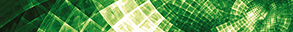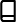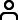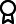Journal metrics
See full report
Acceptance rate41%
Submission to final decision45 days
Acceptance to publication26 days
CiteScore0.900
Journal Citation Indicator1.000
Impact Factor1.555

### Article of the Year 2021

An Application of Sombor Index over a Special Class of Semigroup Graph

####Journal profile

Journal of Mathematics is a broad scope journal that publishes original research and review articles on all aspects of both pure and applied mathematics.

####Editor spotlight

Chief Editor, Professor Jen-Chih Yao, is currently based at Zhejiang Normal University in China. His current research includes dynamic programming, mathematical programming, and operations research.

####Special Issues

We currently have a number of Special Issues open for submission. Special Issues highlight emerging areas of research within a field, or provide a venue for a deeper investigation into an existing research area.

## Latest Articles

More articles
Research Article

### An Overview of Composite Standard Elastic-Net Distribution Based on Complex Wavelet Coefficients

Statistical tools have changed significantly in the past decade; when the maximum likelihood method is usually applied, it provides an inaccurate solution due to its unsuitable properties and causes problems in fit. However, the current Ordinary Least Square (OLS) Model is more reliable in specific situations where the estimation is based on the slope only. On the other hand, some methods depend on the slope, and the intercept is recommended. These methods can be described as thresholding rules. Another often preferred method is the posterior mean (PM) technique. This procedure depends on two parts, the first part is the likelihood, and the other is the prior distribution, where the second part plays a significant role in the estimation. In this article, the standard elastic-net distribution is assumed as the prior part, which consists of two parts, the first being the normal distribution and the second being the double exponential distribution. The reason why this model is used is that wavelet tools have different levels of resolution. Thus, this model may provide a more accurate estimation for the wavelet coefficients, which might be estimated using a normal or double exponential distribution. In the past, some properties of elastic-net penalized were introduced and discussed. However, more properties are introduced for this distribution. In addition, two models based on the elastic-net method are demonstrated, involving the point mass prior. The first model combines normal as likelihood, and elastic-net distributions as prior, while the other combines the double exponential distribution as likelihood with the elastic-net distribution as prior. Moreover, the level-dependent components are estimated at each resolutions. A simulated investigation is studied using the Markov Chain Monte Carlo (MCMC) tool to estimate the underlying features, where real data are involved and modelled using the proposed methods. A stationary wavelet basis is also applied. As a result, the proposed procedure reduces noise levels which may be helpful since noise levels often corrupt real data, usually a significant cause of most numerical estimation problems.

Research Article

### Decomposition Methods for Solving Finite-Horizon Large MDPs

Conventional algorithms for solving Markov decision processes (MDPs) become intractable for a large finite state and action spaces. Several studies have been devoted to this issue, but most of them only treat infinite-horizon MDPs. This paper is one of the first works to deal with non-stationary finite-horizon MDPs by proposing a new decomposition approach, which consists in partitioning the problem into smaller restricted finite-horizon MDPs, each restricted MDP is solved independently, in a specific order, using the proposed hierarchical backward induction (HBI) algorithm based on the backward induction (BI) algorithm. Next, the sub-local solutions are combined to obtain a global solution. An example of racetrack problems shows the performance of the proposal decomposition technique.

Research Article

### New Class of Close-to-Convex Harmonic Functions Defined by a Fourth-Order Differential Inequality

In the recent past, various new subclasses of normalized harmonic functions have been defined in open unit disk U which satisfy second-order and third-order differential inequalities. Here, in this study, we define a new class of normalized harmonic functions in open unit disk U which is satisfying a fourth-order differential inequality. We investigate some useful results such as close-to-convexity, coefficient bounds, growth estimates, sufficient coefficient condition, and convolution for the functions belonging to this new class of harmonic functions. In addition, under convex combination and convolution of its members, we prove that this new class is closed, and we also give some lemmas to prove our main results.

Research Article

### On Reverse Valency Based Topological Characterization of a Chemical Compound

Metal-organic frameworks explicit the consequence of these frameworks with adjustable implementations, namely, energy storage gadgets of magnificent electrode materials, gas store, heterogeneous catalysis, environmental hazard, estimation of chemicals, recognizing of definite gases, controlling solids, and supercapacitors. In this paper, we give explicit expression of the reverse general Randic index, the reverse atom bond connectivity index, the reverse geometric arithmetic index, the reverse forgotten index, the reverse Balaban index, the reverse augmented index, and different types of reverse Zagreb indices of the metal-organic framework M1TPyP-M2 (TPyP = 5, 10, 15, 20-tetrakis (4-pyridyl) porphyrin and M1, M2 = Fe and Co). A graphical comparison of the calculated different types of the reverse degree based topological indices with the aid of the numerical values is also included.

Research Article

### Asymmetric Exchange Rate Pass-Through to Consumer Prices in Ghana: Evidence EMD-NARDL Approach

This paper analyses the US dollar exchange rate pass-through to consumer prices in Ghana from January 1990 to January 2020 using the empirical mode decomposition-based nonlinear autoregressive distributed lags model (EMD-NARDL). This model eliminates the noise component of the underlying data and captures the short- and long-run nonlinearities. We find evidence of cointegration between denoised series of consumer prices and exchange rate and asymmetric pass-through in both the short- and long-run. Specifically, exchange rate pass-through was found to be in the long-run incomplete in the period of depreciation and statistically zero pass-through in the period of appreciation. In the short-run, the exchange rate pass-through in periods of depreciation is near complete; that is, 81% against 74% in periods of appreciation. We recommend that monetary authorities consistently monitor exchange rate behaviour and maintain efficient exchange rate management policies to ensure stable consumer prices. This could be achieved through proper and timely policy interventions using the available monetary policy tools such as foreign exchange reserves.

Research Article

### Location of the Zeros of Certain Complex-Valued Harmonic Polynomials

Finding the approximate region containing all the zeros of analytic polynomials is a well-studied problem. But the number of the zeros and regions containing all the zeros of complex-valued harmonic polynomials is relatively a fresh research area. It is well known that all the zeros of analytic trinomials are enclosed in some annular sectors that take into account the magnitude of the coefficients. Following Kennedy and Dehmer, we provide the zero inclusion regions of all the zeros of complex-valued harmonic polynomials in general, and in particular, we bound all the zeros of some families of harmonic trinomials in a certain annular region.Journal metrics
See full report
Acceptance rate41%
Submission to final decision45 days
Acceptance to publication26 days
CiteScore0.900
Journal Citation Indicator1.000
Impact Factor1.555Author guidelinesEditorial boardDatabases and indexingAuthor guidelinesEditorial boardDatabases and indexing

Article of the Year Award: Outstanding research contributions of 2021, as selected by our Chief Editors. Read the winning articles.